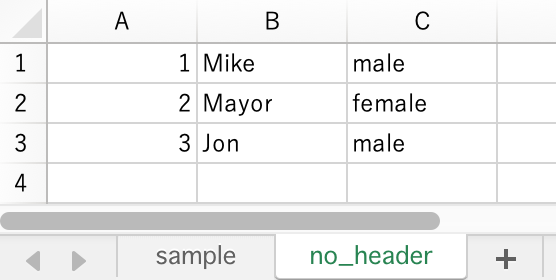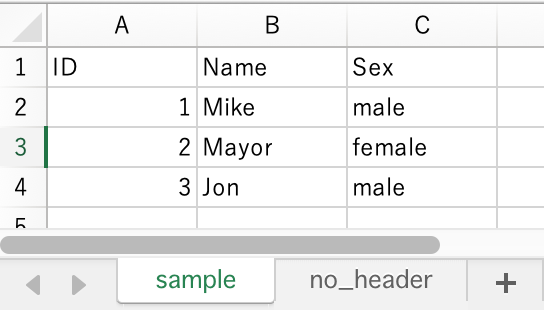# Use openpyxl - Convert to DataFrame in Pandas

Page content

## Introduction

Previously, I wrote several articles on working Excel files using openpyxl.

In this article, I introduce how to convert openpyxl data to Pandas data format called `DataFrame`.

## Preparation

### Install modules

First, install module with `pip` command.

``pip install openpyxl pandas``

### Make sample data

Second, create a new file named `sample.xlsx` including the following data.

• Workbook has a sheet named `no_header` that doesn’t have header line.• Workbook has a sheet named `sample` that has a header line.## Convert openpyxl object to DataFrame

Load Excel data with openpyxl and convert to `DataFrame`. `DataFrame` is used to represent 2D data on Pandas. Since Excel data is also 2D data expressed by rows and columns, `Worksheet` object in [openpyxl] (https://openpyxl.readthedocs.io/en/stable/index.html) can be converted to Pandas` DataFrame` object.

When converting a file that has no header line, give `values` property on `Worksheet` object to `DataFrame` constructor.

`````` 1from openpyxl import load_workbook
2import pandas as pd
3
8
9# Convert to DataFrame
10df = pd.DataFrame(ws.values)``````

Check the result.

``1df.head(3)``
012
01Mikemale
12Mayorfemale
23Jonmale

The column number is displayed as a header.

In case of a file that has a header line, it is necessary to change processing. When creating a `DataFrame` object, specify column name with `columns` option. At this time, the length of array given `columns` option must be equal to length of columns in `DataFrame`.

The sample code is as follows.

`````` 1from openpyxl import load_workbook
2import pandas as pd
3
5ws = wb['sample']
6
7data = ws.values
8# Get the first line in file as a header line
9columns = next(data)[0:]
10# Create a DataFrame based on the second and subsequent lines of data
11df = pd.DataFrame(data, columns=columns)``````

Check the result.

``1df.head(3)``
IDNameSex
01Mikemale
12Mayorfemale
23Jonmale

The column name is displayed.

Using the `read_excel` function in Pandas, we can do the same processing. To use `read_excel` function, install xlrd and openpyxl.

``1pip install openpyxl pandas xlrd``

Call `read_excel` function as below.

``````1import pandas as pd
2
• Convert `Worksheet` object with or without headers to `DataFrame` object
• Make it simpler with `read_excel` function in Pandas